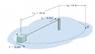# Mass rotating but with string that's decreasing in length over time: work done

jamiebellend
Homework Statement:
Imagine an object attached to a string kept in circular motion about a fixed point [see picture]. How much work is done between the points rA and rB
Relevant Equations:
W=F dot D
For the displacement, how do I figure out the angle theta between the points? And how does the speed at which the string retracts affect the centripetal force?

#### Attachments

•Capture.PNG
11.9 KB · Views: 62
Last edited:

Gold Member
Hi. Pulling code does not affect angular momentum of the block around the center pole. Conservation of angular momentum tells you speed and kinetic energy increased by work done of the block at B.

Homework Helper
Gold Member
2022 Award
Faults in question:
1) Not circular motion - motion will be spiral because r is changing.
2) You are (probably) being asked for the work done by whatever is pulling-in the string; this should have been made clear.

The displacement, angle and centripetal force are not needed (unless you want to make the calculation really hard).

Q1: Can you find the tangential speed at point B? (hint: conservation of angular momenetum)?
Q2: Can you find the increase in the mass's kinetic energy going from A to B?
Q3: How is the work done related to the answer to Q2?

I'm going to bed now (2:21am here in the UK), so won't be posting further for a few hours.